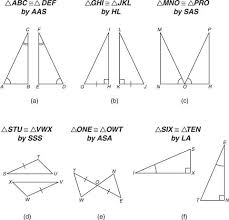Mathematic

# Define and Discuss on Congruent TrianglesBroad objective of this article is to Define and Discuss on Congruent Triangles. Here explain Congruent Triangles in mathematical point of view. Triangles that have precisely the same size and shape are called congruent triangles. The symbol for congruent is usually ≅. Two triangles are congruent if the three sides and the three angles of a single triangle have the exact same measurements as three features and three angles associated with another triangle. Here also discuss on Corresponding parts and Tests for congruence.